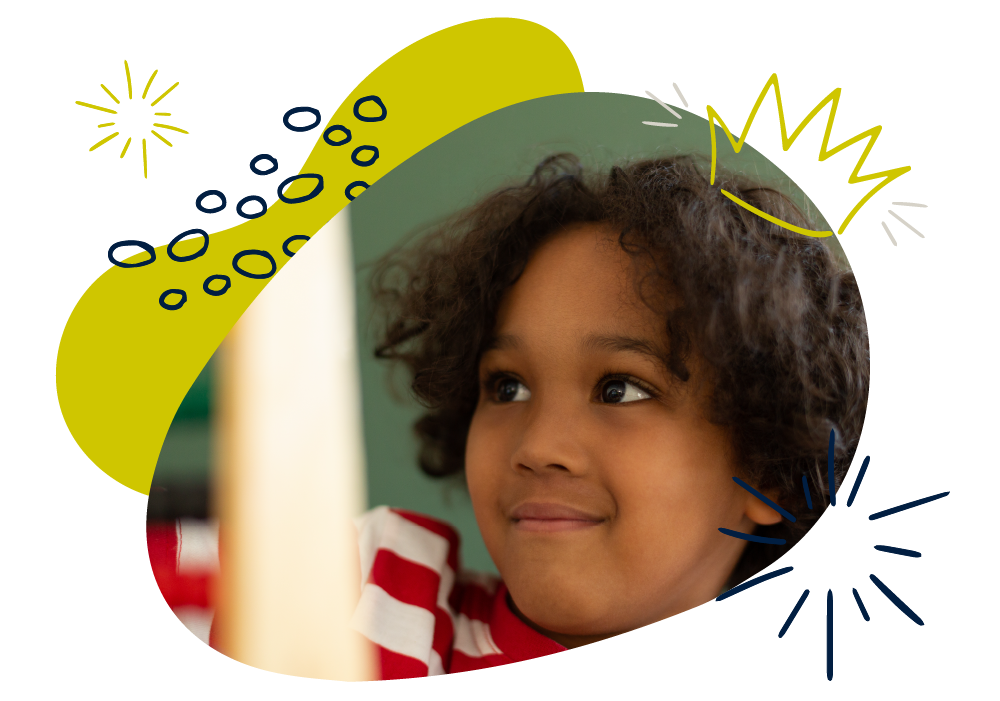We use cookies to enhance your experience on our website. By continuing to use our website, you are agreeing to our use of cookies. You can change your cookie settings at any time. Find out more# Maths: Age 6–7 (Year 2)

In Year 2, your child will continue to develop their counting and calculation skills, learning different ways to multiply and divide. They will also extend their understanding of fractions. They will measure length, weight, volume, temperature, time and money, discover more about 2D and 3D shapes, and begin to learn about statistics.

They’ll count in steps of 2, 3, 5 and 10 and learn number bonds to 20. They’ll partition numbers into 10s and 1s to add and subtract one-digit and two-digit numbers. They will be taught the 2, 5 and 10 times tables and how to find , , , of quantities and shapes.

Much of their learning will come from exploring with objects to solve problems practically and there are simple things you can do at home to support their development.

## How to help at home

You don’t need to be an expert to support your child with maths or help them develop a good sense of number! Here are three simple, but effective learning ideas that you can try with your child using everyday items at home.

### 1. Partition numbers

Partitioning means to break numbers into parts. Use objects, such as straws grouped in tens, to show numbers split into tens and ones. How many ways can your child find to partition a number?

For example, 54 could be 50 + 4, 40 + 14, 30 + 24, 20 + 34, or 10 + 44.

### 2. Tell stories

Make up addition and subtraction stories together. For example: Two badgers, three hedgehogs and a toad have a picnic. How many animals are there altogether? Four rabbits join in. How many are there now? Two animals go home. How many are left?

### 3. Play shops

To help your child get used to the value of different coins and notes, use real money to play shops. Price up some toys and take turns to be the customer who pays and the shopkeeper who works out the change.

You’ll find more activity ideas in our Kids’ activities section.

## What your child will learn at school

#### Number & place value in Year 2 (age 6–7)

In Year 2, children will be expected to recognise tens and ones in 2-digit numbers (for example 23 has 2 tens and 3 ones) and use these to order numbers to 100. This includes:

• counting in steps of 2, 3, 5 and 10
• using more than (>), less than (<) and equals (=) symbols to compare numbers
• using place value and number facts to solve problems.

#### Addition & subtraction in Year 2 (age 6–7)

In Year 2, children will be expected to be able to solve addition and subtraction problems using numbers with one and two digits. This includes:

• knowing and using addition and subtraction facts up to 20 and working out related addition and subtraction facts to 100
• adding and subtracting numbers using objects, pictures and drawings, and also solving these problems mentally
• understanding that addition and subtraction have an inverse relationship, i.e. they undo each other, and using this to check their calculations.

#### Multiplication & division in Year 2 (age 6–7)

In Year 2, children will be expected to use a range of methods to solve multiplication and division problems, including using practical resources and mental methods. This includes:

• knowing and using multiplication and division facts for the 2, 5 and 10 times tables
• recognising and identifying odd and even numbers
• using the symbols ×, ÷ and = to record multiplication and division calculations.

#### Fractions in Year 2 (age 6–7)

In Year 2, children will be expected to be able to recognise and use the fractions  ,  and . This includes:

• finding fractions of lengths, shapes, sets of objects and quantities
• writing simple fractions, such as  of 6 = 3
• recognising that  and  mean the same.

#### Geometry in Year 2 (age 6–7)

In Year 2, children will be expected to be able to compare and sort common 2D and 3D shapes and everyday objects and continue patterns of shapes. This includes:

• describing 2D shapes including number of sides and lines of symmetry
• describing 3D shapes including number of faces, edges and vertices
• describing turns using clockwise, anti-clockwise and right angles.

#### Measurement in Year 2 (age 6–7)

In Year 2, children will be expected to use standard units to measure length, mass (weight), capacity and temperature, compare intervals of time and solve simple problems with money. This includes:

• comparing and ordering measures such as metres (m), centimetres (cm), kilograms (kg), grams (g), degrees Celsius (°C), litres (l) and millilitres (ml)
• telling the time to five minutes, including quarter to and quarter past
• choosing coins or notes to make a given amount of money and working out change.